Next: Markov Decision Processes with Up: -MDPs: Learning in Varying Previous: Introduction

# Preliminaries

To begin with, we recall the definition of a Markov Decision Process (MDP) [Puterman(1994)]. A (finite) MDP is defined by the tuple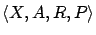, where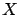anddenotes the finite set of states and actions, respectively.is called the transition function, since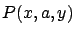gives the probability of arriving at state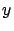after executing action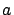in state. Finally,is the reward function,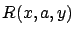gives the immediate reward for the transition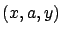.

Subsections